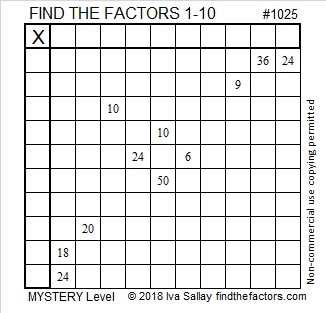# 1025 Mystery Date

It is a mystery why we in the United States write our dates “month-day-year”. It makes about as much sense as saying larger, large, largest or better, good, best. Nevertheless, it is what it is.

So today in the United States it is 2-7-18, the e-day of the century. It’s not quite as exciting as 2-7-1828 might have been, but still pretty exciting. e is also known as Euler’s number, and like pi, it is an irrational number. A college professor of mine taught me how to remember its first few digits by remembering 2.7, the year 1828 twice, and 45-90-45 (that very important isosceles triangle). Thus, e ≈ 2.718281828459045.

The difficulty level of today’s puzzle is also a mystery. Nevertheless, you can still solve it by applying logic and facts from a simple 10×10 multiplication table:Print the puzzles or type the solution in this excel file: 10-factors-1019-1027

Here are a few facts about the number 1025:

1025 can be written as the sum of consecutive prime numbers two different ways:
97 + 101 + 103 + 107 + 109 + 113 + 127 + 131 + 137 = 2025; that’s nine consecutive prime numbers.
71 + 73 + 79 + 83 + 89 + 97 + 101 + 103 + 107 + 109 + 113 = 2025; that’s eleven consecutive prime numbers.

1025 is the sum of two squares three different ways:
25² + 20² = 1025
32² + 1² = 1025
31² + 8² = 1025

That previous fact contributes to the fact that 1025 is the hypotenuse of SEVEN Pythagorean triples:
64-1023-1025 calculated from 2(32)(1), 32² – 1², 32² + 1²
225-1000-1025 which is 25 times (9-40-41) and can also be calculated from 25² – 20², 2(25)(20), 25² + 20²
287-984-1025 which is (7-24-25) times 41
420-935-1025 which is 5 times (84-187-205)
496-897-1025 calculated from 2(31)(8), 31² + 8², 31² + 8²
615-820-1025 which is (3-4-5) times 205
665-780-1025 which is 5 times (133-156-205)

1025 is also a wonderful palindrome in three different bases.
10000000001 in BASE 2
100001 in BASE 4
101 in BASE 32

• 1025 is a composite number.
• Prime factorization: 1025 = 5 × 5 × 41, which can be written 1025 = 5² × 41
• The exponents in the prime factorization are 2 and 1. Adding one to each and multiplying we get (2 + 1)(1 + 1) = 3 × 2  = 6. Therefore 1025 has exactly 6 factors.
• Factors of 1025: 1, 5, 25, 41, 205, 1025
• Factor pairs: 1025 = 1 × 1025, 5 × 205, or 25 × 41
• Taking the factor pair with the largest square number factor, we get √1025 = (√25)(√41) = 5√41 ≈ 32.01562